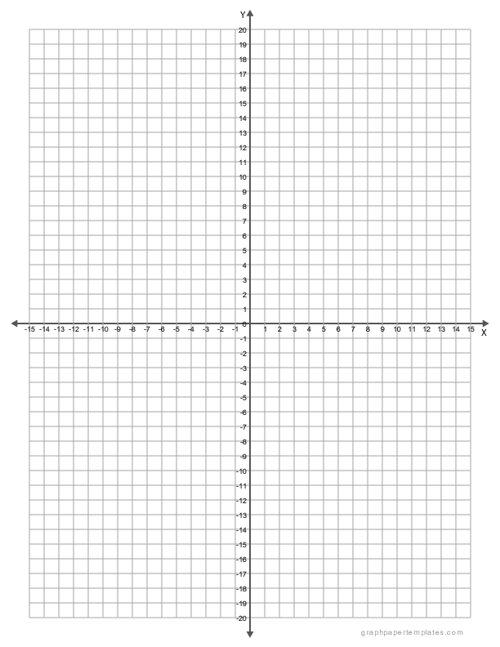# Coordinate Graph PaperPaper Size: Letter

Format: PDF

Size: 8.5" x 11"  -  ( 216 mm x 279 mm )

Layout: Portrait / Vertical

Color: Gray

Coordinate graph paper can be a handy tool for a variety of tasks, from plotting the trajectory of a projectile to helping students visualize the properties of geometric shapes.

## Printable Coordinate Graph Paper PDF

The paper is divided into a grid of small squares, with each square representing a unit of measurement. This makes it easy to plot points and track measurements, which can be helpful when working with complex data sets.

Coordinate graph paper can also be used for educational purposes, such as teaching students the basics of graphing or helping them visualize the relationships between different variables.

In addition, the paper can be used for art projects or as a template for creating patterns. Whether you're a student, teacher, or professional, coordinate graph paper can be a useful tool for a variety of tasks.

## Features of Coordinate Graph Paper Printable PDF:

• Graph paper with 1/4 inch line spacing;
• Comes with X-axis and Y-axis;
• X-axis and Y-axis numbered from -15 to 15 and -20 to 20 respectively;

Coordinate Graph Paper

### You may also like:

3D Isometric Graph Paper (Blue)

3D Isometric Graph Paper, with lines at 60 degrees and blue in color. It is perfect for creating three-dimensional images.

Isometric Graph Paper

Isometric graph paper is a sort of graph paper with each line forming a 60-degree angle. It creates a 3D effect and can be used for isometric illustrations or designs. Download and print today!

Cornell Notes Template

The Cornell Notes Template is useful for taking notes on any type of lecture material, and it is especially effective for classes that require a lot of memorization.

Engineering Graph Paper

Engineering graph paper is a type of graph paper with horizontal and vertical lines at equal distance with major lines appearing at a specified distance of 1/2 inch, 1/4th, 1/5th, 1/10th, or every 5 or 10 lines.

1/8" Inch Graph Paper

Download free printable 1/8" inch graph paper with blue grid lines in portrait orientation. It comes with 1/8 inch squares so it is perfect for students to use for their math, graphing, and designing projects.